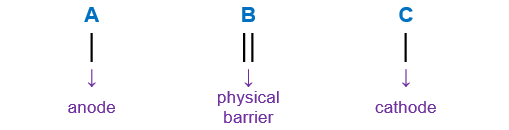# Problem: In a zinc-copper cell, Zn | Zn2+ (1M) | | Cu2+ (1M) | Cu, which electrode is negative?

###### FREE Expert Solution

We’re being asked to identify the negative electrode in the following in a zinc-copper cell:

Zn | Zn2+ (1M) | | Cu2+ (1M) | Cu

When writing a cell notation, we use the following format – “as easy as ABCRecall the mnemonics LEO GER.

81% (399 ratings)###### Problem Details

In a zinc-copper cell, Zn | Zn2+ (1M) | | Cu2+ (1M) | Cu, which electrode is negative?

Frequently Asked Questions

What scientific concept do you need to know in order to solve this problem?

Our tutors have indicated that to solve this problem you will need to apply the Cell Notation concept. If you need more Cell Notation practice, you can also practice Cell Notation practice problems.